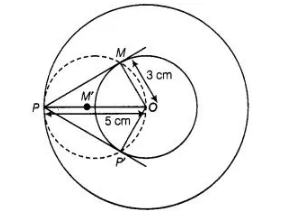# Draw two concentric circles of radii 3 cm and 5 cm.

Question:

Draw two concentric circles of radii 3 cm and 5 cm. Taking a point on outer circle construct the pair of tangents to the other. Measure the length of a

tangent and verify it by actual calculation.

Solution:

Given, two concentric circles of radii 3 cm and 5 cm with centre 0. We have to draw pair of

tangents from point P on outer circle to the other.Steps of construction

1. Draw two concentric circles with centre 0 and radii 3 cm and 5 cm.
2. Taking any point P on outer circle. Join OP.
3. Bisect OP, let M’ be the mid-point of .
Taking M’ as centre and OM’ as radius draw a circle dotted which cuts the inner circle at M and P’.
4. Join P M and PP’. Thus, PM and PP’ are the required tangents.
5. On measuring PM and PP’, we find that PM = PP’ = 4 cm.

Actual calculation

In right angle $\triangle O M P$, $\angle P M O=90^{\circ}$

$\therefore$ $P M^{2}=O P^{2}-O M^{2}$

[by Pythagoras theorem i.e. (hypotenuse) $^{2}=(\text { base })^{2}+$ (perpendicular) $^{2}$ ]

$\Rightarrow \quad P M^{2}=(5)^{2}-(3)^{2}=25-9=16$

$\Rightarrow \quad P M=4 \mathrm{~cm}$

Hence, the length of both tangents is $4 \mathrm{~cm}$.